Heat Exchangers - 4

# Heat Exchangers - 4 Notes | Study Heat Transfer - Mechanical Engineering

## Document Description: Heat Exchangers - 4 for Mechanical Engineering 2022 is part of Heat Exchangers for Heat Transfer preparation. The notes and questions for Heat Exchangers - 4 have been prepared according to the Mechanical Engineering exam syllabus. Information about Heat Exchangers - 4 covers topics like and Heat Exchangers - 4 Example, for Mechanical Engineering 2022 Exam. Find important definitions, questions, notes, meanings, examples, exercises and tests below for Heat Exchangers - 4.

Introduction of Heat Exchangers - 4 in English is available as part of our Heat Transfer for Mechanical Engineering & Heat Exchangers - 4 in Hindi for Heat Transfer course. Download more important topics related with Heat Exchangers, notes, lectures and mock test series for Mechanical Engineering Exam by signing up for free. Mechanical Engineering: Heat Exchangers - 4 Notes | Study Heat Transfer - Mechanical Engineering
 1 Crore+ students have signed up on EduRev. Have you?

Lecture 35 - Heat Exchangers, Heat Transfer

8.2.4 Why multi-pass exchangers?
The simplest type of heat exchangers is double pipe heat exchangers, which is inadequate for flow rates that cannot readily be handled in a few tubes. If several double pipes are used in parallel, the metal weight required for the outer tubes becomes so large that the shell and tube construction, such as 1-1 exchanger will be helpful. In that one shell serves for many tubes, is economical. The heat transfer coefficient of tube side and shell side fluid is very important and the individual heat transfer coefficients must be high enough to attain high overall heat transfer coefficient. As the shell would be quite large as compared to the tubes, the velocity and the turbulence of the shell side fluid is important.

In contrast, the 1-1 exchanger has limitations also. When the tube side flow is divided evenly among all the tubes, the velocity may be quite low, resulting in low heat transfer coefficient. There it may be required to increase the area to have the desired heat exchange for this low heat transfer coefficient. The area may be increased by increasing the length of the tube. However, the tube length requirement may be impractical for a given situation. Thus the number of tubes should be increased without increased the tube length. The increased number of tubes would also provide the increased velocity in the shell side resulting in the higher heat transfer coefficient. Therefore, multi-pass construction is needed, which would permit to use the practical and standard tube lengths. However, the disadvantages are that,

1. The construction of the exchangers become complex.
2. Parallel flow cannot be avoided.
3. Additional friction losses may occur.

It should be noted that generally even number of tube passes are used in multi pass exchanger.

8.2.5 LMTD correction factor

In the earlier chapter, we have seen for co-current or counter current flow system. The average driving force for heat transfer was defined by log mean temperature difference (LMTD). Thus the LMTD can be used for 1-1 exchangers for co-current and counter current. However, for multi pass exchangers (1-2, 2-4, etc.) the fluids are not always in co-current or counter current flow.  The deviation for co-current or counter current flow causes a change in the average driving force. Therefore, in order to use true heat transfer driving force, a correction factor is required into the LMTD. Thus, the heat transfer rate can be written as (eq.8.8),

q = UdA(FTΔTm)                  (8.8)

where,

Ud = overall heat transfer coefficient including fouling/dirt

A = heat transfer area

FΔTm = true average temperature difference.

FT = LMTD correction factor

It is to be noted that the following assumption have been considered for developing LMTD,

1. The overall heat transfer coefficient is constant throughout the exchanger
2. In case any fluid undergoes for phase change (e.g., in condenser), the phase change occurs throughout the heat exchanger and the constant fluid temperature prevails throughout the exchanger.
3. The specific heat and mass flow rate and hence the heat capacity rate, of each fluid is constant.
4. No heat is lost in to the surroundings.
5. There is no conduction in the direction of flow neither in the fluids nor in the tube or shell walls.
6. Each of the fluids may be characterized by a single temperature, at any cross section in the heat exchanger that is ideal transverse mixing in each fluid is presumed.

FT, the LMTD correction factor can be directly obtained from available charts in the literature. These charts were prepared from the results obtained theoretically by solving the temperature distribution in multi-pass heat exchangers.

Figures 8.12 and 8.13 show the two generally used heat exchangers and their corresponding plots for finding FT. It may be noted that the given figures have the representative plots and any standard book on heat transfer may be consulted for the accurate results.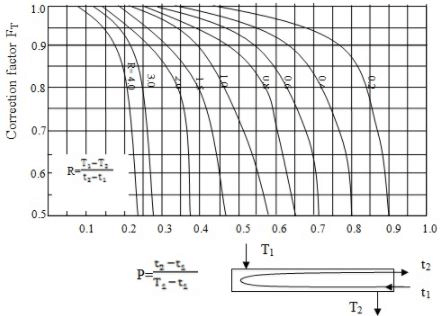Fig. 8.12: FT plot for 1-2 exchanger; t: cold fluid in the tube; T: hot fluid in the shell; 1: inlet; 2: outlet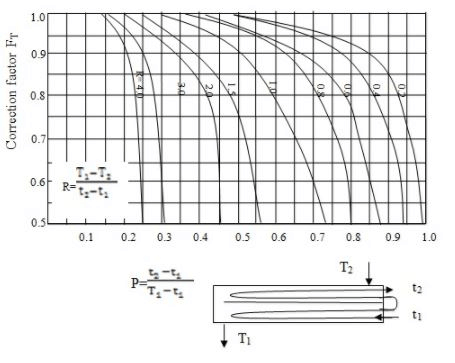Fig. 8.13: FT plot for 2-4 exchanger; t: cold fluid in the tube; T: hot fluid in the shell; 1: inlet; 2: outlet

It should be noted that in case of condensation or evaporation the correction factor becomes unity (FT =1). While designing a heat exchanger, the rule of thumb is that the FT should not be less than 0.8.

8.2.6 Individual heat transfer coefficient
In section 8.2.1, we have seen that the overall heat transfer coefficient can be calculated provide the parameters are known including individual heat transfer coefficients. In this, section we will discuss how to find out the individual heat transfer coefficient, which is basically based on the well-established correlations and discussed earlier also.

The heat transfer coefficient (hi) for the tube side fluid in a heat exchanger can be calculated either by Sieder-Tate equation or by Colburn equation discussed in earlier chapter.

However, the shell side heat transfer coefficient (ho) cannot be so easily calculated because of the parallel, counter as well as cross flow patterns of the fluid. Moreover, the fluid mass velocity as well as cross sectional area of the fluid streams vary as the fluid crosses the tube bundle. The leakages between baffles and shell, baffle and tubes, short circuit some of the shell fluid thus reduces the effectiveness of the exchanger.

Generally, modified Donohue equation (eq.8.9)  is used to predict the ho,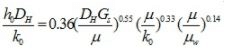(8.9)

where,

h0= shell side heat transfer coefficient

Dh= hydraulic diameter of the shell side
k0= thermal conductivity of the shell side fluid
Gs= mass flow rate of the shell side

The Dh and Gs can be easily calculated if the geometry of the tube arrangement in the shell is known. The tubes may be generally arranged as a square or triangular pitch, as shown in figure 8.14.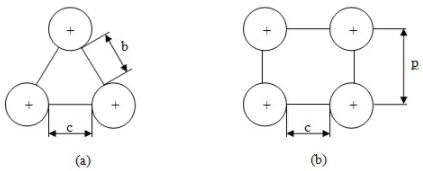Fig.8.14: Tube arrangement in the shell (a) triangular pitch (b) square pitch

The hydraulic diameter (Dh) for tubes on square pitch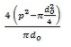Dh For 60° triangular pitch=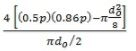where,

d0 = outer diameter of tube

p = tube pitch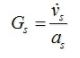where,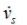= flow rate of shell fluid

as = shell side flow area

Shell side flow area can be calculated using baffle information number of tubes in the shell and tube arrangement. If 25% cut baffles are used, that means the shell side flow will be from this 25% area. However we have to reduce the area of the pipes which are accumulated in this opening. So depending upon the information we may determine the shell side fluid flow area. It may also be found out by the following way,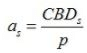where,

C = tube clearance
B = baffle spacing
Ds= inside diameter of shell
p = pitch of the tube

The document Heat Exchangers - 4 Notes | Study Heat Transfer - Mechanical Engineering is a part of the Mechanical Engineering Course Heat Transfer.
All you need of Mechanical Engineering at this link: Mechanical Engineering

## Heat Transfer

58 videos|70 docs|85 tests
 Use Code STAYHOME200 and get INR 200 additional OFF

## Heat Transfer

58 videos|70 docs|85 tests

Track your progress, build streaks, highlight & save important lessons and more!

,

,

,

,

,

,

,

,

,

,

,

,

,

,

,

,

,

,

,

,

,

;